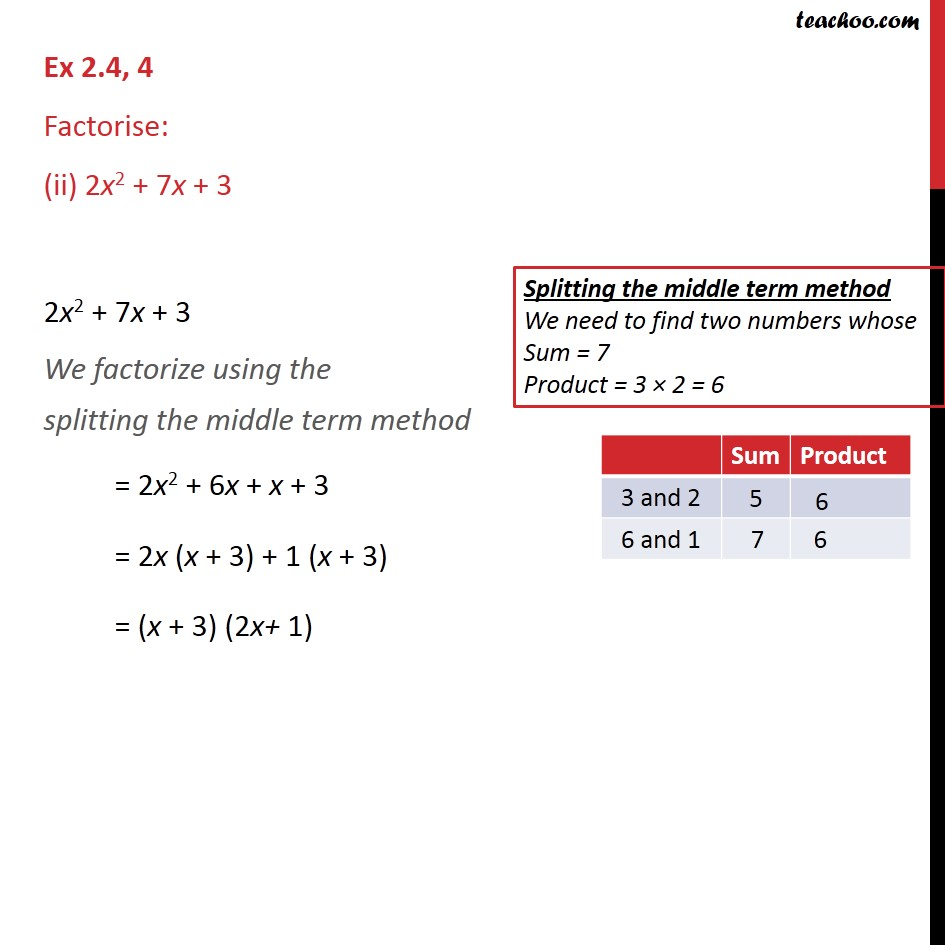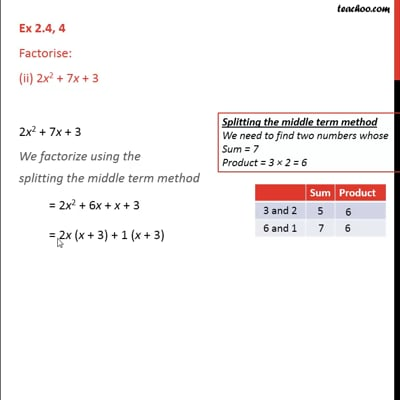Ex 2.4

Chapter 2 Class 9 Polynomials
Serial order wiseThis video is only available for Teachoo black users

Maths Crash Course - Live lectures + all videos + Real time Doubt solving!

### Transcript

Ex 2.4, 4 Factorise: (ii) 2x2 + 7x + 3 2x2 + 7x + 3 We factorize using the splitting the middle term method = 2x2 + 6x + x + 3 = 2x (x + 3) + 1 (x + 3) = (x + 3) (2x+ 1)